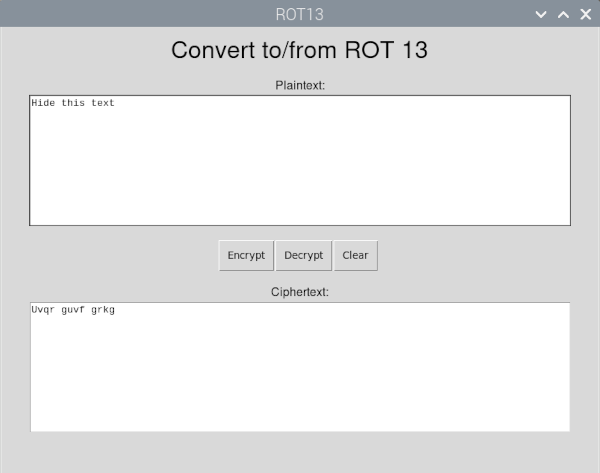# Programming a GUI - Python guizero

Traditionally creating graphical applications (GUI apps) has been hard. It takes a lot to setup and configure all the basics. Fortunately guizero has come along to make it easy to create GUIs in Python. It removes much of the boiler plate code allowing you to concentrate on creating the code to make the application work.

## Understanding GUI apps

GUI is short for graphical user interface, which describes applications that you run in a graphical environment, such as Windows, Linux and Mac. Applications which you typically interact with using a combination of keyboard and mouse, or through touch.

## The guizero library

The guizero library provides an easy way to get started programming a GUI with Python. It removes the need for much of the boiler plate code and makes it easy to create simple applications.

The guizero library is based on TKinter which is the built in GUI environment for Python.

## Cryptography and ROT13 encryption cipher

This is designed as an example project to show how to use guizero, but I’ve based it on something I’ve been doing on my sister website Penguin Fortress. On the penguin fortress youtube channel I’m creating some videos on cryptography and recently discussed ROT13 encryption as a way of obscuring information. Note this is a very week form of encryption. It’s not suitable for any data you want to keep secret, but is useful for hiding the punchline of a joke etc.

To understand about cryptography and about RO13 encryption see the video below.

## The ROT13 GUI app in Python

The application is shown in the image below. It has an area for entering the plaintext, then clicking on Encrypt will populate the ciphertext field. It can also be operated in reverse by entering the ciphertext into the appropriate field and clicking on Decrypt.## Source code for ROT13 Gui

``````

#!/usr/bin/env python3

from guizero import App, Text, TextBox, PushButton, Box

def rot13 (plaintext):

ciphertext = ""

for pt_chr in plaintext:

# Check if it's an alpha character (otherwise leave unchanged)

# convert to a number

pt_int = ord(pt_chr)

if (pt_int >= ord('A') and pt_int <= ord('Z')):

# it's upper case

ct_int = pt_int + 13

# check to see if we need to wrap around

if ct_int > ord('Z'):

ct_int -= 26

ciphertext += chr(ct_int)

elif (pt_int >= ord('a') and pt_int <= ord('z')):

# it's  lower case

ct_int = pt_int + 13

# check to see if we need to wrap around

if ct_int > ord('z'):

ct_int -= 26

ciphertext += chr(ct_int)

else:

# not alpha char so just add

ciphertext += pt_chr

return ciphertext

def encrypt():

encrypted_text = rot13 (plaintext_text.value)

ciphertext_text.value = encrypted_text

def decrypt():

decrypted_text = rot13 (ciphertext_text.value)

plaintext_text.value = decrypted_text

def clear_text():

plaintext_text.value = ""

ciphertext_text.value = ""

app = App(title="ROT13", width=800, height=600)

title = Text(app, text="Convert to/from ROT 13", size=24, height=2)

plaintext_title = Text(app, text="Plaintext:")

plaintext_text = TextBox(app, width=90, height=10, multiline=True)

buttons_box = Box(app, height=80, width=220)

# Buttons are contained within the Box

encrypt_button = PushButton(buttons_box, text="Encrypt", align="left", command=encrypt)

decrypt_button = PushButton(buttons_box, text="Decrypt", align="left", command=decrypt)

clear_button = PushButton(buttons_box, text="Clear", align="left", command=clear_text)

ciphertext_title = Text(app, text="Ciphertext:")

ciphertext_text = TextBox(app, width=90, height=10, multiline=True)

app.display()

``````